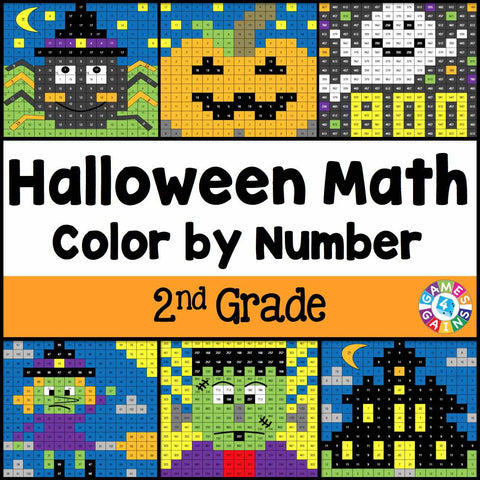## Halloween Math Color-by-Number - 2nd Grade

• \$480

Halloween Math Color-by-Number set comes with 6 Halloween math color-by-number activities for reviewing 2nd grade math skills. This Halloween math set is perfect to use in centers, in small groups, or with the whole class!

Included with this Halloween Math Color-by-Number set:
Instructions
6 color by number worksheets
6 student problem worksheets
6 teacher answer sheets

Here are the skills covered by these Halloween math color-by-number activities:

• Finding the missing number in number bonds to 20
• Finding the unknown in addition problems to 20
• Solving one-step addition and subtraction words problems to 20
• Determining the number from base 10 models
• Interpreting numbers written in word from unit form, word form, and expanded form
• Regrouping numbers (i.e. 3 tens and 14 ones = 44)

Want to learn more about what you'll get? Check out the preview!